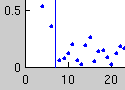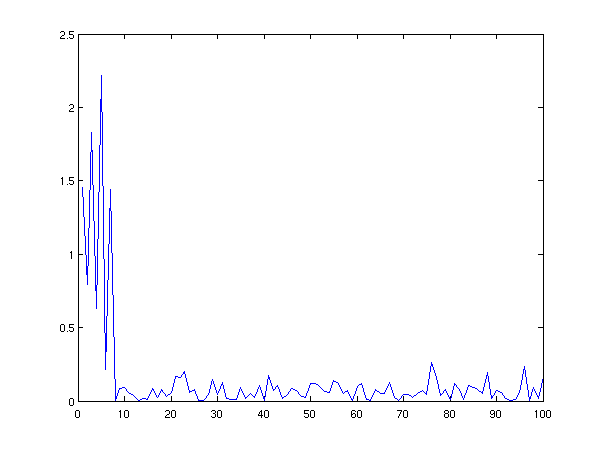## Overviews### Twimpact

Micro-blogging services like twitter have become very popular in recent years. As of now, exploring such services and finding interesting users is still an open problem.

With our project twimpact we explore methods for automatically measuring user impact, or studying the network structure of micro-blogging services.### On Relevant Dimensions in Kernel Feature Spaces

It is well known that using a kernels for supervised learning corresponds to mapping the problem into a potentially quite high-dimensional feature space. Usually, complexity control (for example, large margin hyperplanes) is attributed with the fact that learning is still possible. We showed, however, that using the right kernel leads to a typically small effective dimensionality in a feature space, meaning that even in infinite-dimensional feature spaces, the learning problem is in fact contained in a low-dimensional subspace.

There is software available for matlab and R.

## Twimpact

Joint work with Matthias L. Jugel (a.k.a. Leo).

November 20, 2009

Micro-blogging services like twitter have become very popular in recent years. As of now, exploring such services and finding interesting users is still an open problem.

With our project twimpact we explore methods for automatically measuring user impact, or studying the network structure of micro-blogging services.

Currently, we are crawling twitter for so-called "Retweets", and compute user impact from that. In addition, we perform real-time retweet trending.

## On Relevant Dimensions in Kernel Feature Spaces

Joint work with Joachim Buhmann, Klaus-Robert Müller.

November 15, 2008

The kernel feature space always plays a somewhat obscure role in kernel machines. What you generally learn about this space is that it is pretty high-dimensional (even infinite dimensional), and that you wouldn't want to deal with it explicitly anyway because you can directly compute scalar products using the kernel function.

There is nothing fundamentally wrong with this statement. However, there are some interesting insights you can get about the kernel feature space. Only because you wouldn't want to deal with the kernel feature space directly computationally does not mean that you cannot study it theoretically.

In fact, it turns out the usual picture that the data in the kernel feature space is inherently high-dimensional is in general wrong. As we discuss in our paper "On the Relevant Dimensionality in Kernel Feature Spaces", it turns out that the relevant information about a supervised learning problem (meaning the information necessary to learn well) is contained in a comparatively low-dimensional subspace of the kernel feature space.

The dimensionality of this subspace is characterized by the interplay between the data set and the used kernel, and it typically is also much smaller than the trivial "maximal" dimensionality of the data set given by the number of samples (because n points usually lie in a subspace of dimension n).

The tool we have employed in our paper is kernel principal component analysis, that is, the usual principal component analysis method in feature space which identifies orthogonal directions which maximize the variance of the data.

As it turns out, one can prove that the information about X and Y is contained in a finite-dimensional subspace whose dimensionality does also not grow with the number of samples. As a consequence, the regularization typically employed in conjunction with kernel methods works because the data is contained in a low-dimensional subspace with large variance, and the regularization ensures that the learned predictor focusses on this subspace.

For more information, have a look at the full paper. There is also some software available in matlab which also contains an estimator of the relevant dimensionality, as well as a package for R written by our student Jan Müller.

An Example

For starters, it is actually pretty simple to validate what I have claimed above for a concrete data set. Using the kernels implemented in the software package mentioned above, we can play around a bit with some toy data to illustrate the point I made above. If you don't want to type in the commands yourself, you can the code below in a single matlab file (including more code to put sensible labels on the axes.)

First, let us generate some data, the noisy sinc data set

```[X, Y] = sincdata(100, 0.1);
```

If you want, you can plot the dataset

```plot(X, Y, '.')
```Next, we construct a kernel matrix

```K = rbfkern(1, X); % 1 is a reasonable width for this data set.
```

The next step is to compute the kernel PCA. You need to know three things about kernel PCA: (1) for normal PCA you compute the eigendecomposition of the covariance matrix, for kernel PCA, you take the kernel matrix. (2) You cannot really compute the principal directions as these are vectors in the feature space (remember, "high-dimensional"). Instead you compute scalar products with these principal directions (so to say, the projections onto the principal directions). If you do this with each data point, you exactly obtain an eigenvector of the kernel matrix.

```[U, D] = sortedeig(K); % my special function which takes
% care of proper sorting.
```

Now, let us first have a look at the eigenvalues which correspond to the variances along the kernel PCA directions.

```plot(diag(D))
```What we see is that there are only a few directions where we have any variance at all, meaning that X is contained already in a low-dimensional subspace in the sense that we can project to this subspace without distorting the distances between the points significantly.

But a different question is how the information about Y relates to the kernel PCA directions. We can study this relationship by forming the scalar products between the eigenvectors of the kernel matrix and the vector containing the corresponding samples from Y.

In order to understand what these scalar products represent, keep in mind that the eigenvectors form an orthonormal (that is, orthogonal and unit length) basis of the n-dimensional vector space (where n is the number of data points), such that scalar products just compute the new coefficients after a basis change. Furthermore, recall that the eigenvectors are the projections of the X samples onto the kernel PCA directions. Therefore, the scalar products compute the contribution of the kernel PCA directions to the label vector.

Let us plot the absolute values of these contributions:

```plot(abs(Y*U)) % Plots contributions vs. decreasing variance
% along kernel PCA directions
```At first, it seems that there actually is some information even in directions where X in feature space has very little variance. On the other hand, we also see that the information seems to be somewhat concentrated in the leading kernel PCA components.

However, the question is if all kernel PCA components are equally relevant for the learning problem. How could directions not contain relevant information? Well, recall that Y is contaminated by noise (in our case additive Gaussian noise), and if a direction contains only contributions from the noise, then we can safely discard that dimension without losing any information.

Okay, we need set up things a bit differently. Let us again construct a data set but this time keep track of the target function and the noise:

```[X, F] = sincdata(100, 0); % note that the noise is zero
N = 0.1 * randn(1, 100);   % the noise
Y = F + N;                 % adding noise manually
K = rbfkern(1, X);         % new kernel function
[U, D] = sortedeig(K);     % eigendecomposition
```

Now since Y * U = F * U + N * U, we can decompose the contributions by relevant information and noise:

```plot(1:100, abs(F*U), 'r', 1:100, abs(N*U), 'b')
legend('sinc(x)', 'noise')
```We see that the information about the sinc function is really contained in the leading few kernel PCA components, and the later kernel PCA components with the negligible variance only contain information about the noise.

In summary, this means that the relevant information about a supervised learning problem is really contained in a low-dimensional subspace of the kernel feature space, even if the whole feature space is infinite-dimensional. The dimensionality depends on the interplay between the kernel and the data set.

In our paper, we derive theoretical bounds on the contribution of the relevant information to a kernel PCA direction with small variance and show that it decays at essentially the rate of the eigenvalues. We also demonstrate that you can learn on the reduced feature space using a simple (unregularized!) least squared regression method as well as support vector machines on the whole set.

We also present a method for estimating the relevant dimensionality. Based on this estimate, one can explicitly construct the low-dimensional feature space, and also gain further insights into the amount of match between the kernel and the data set. This piece of information can be quite interesting, in particular, when the performance achieved so far is not good. In that case, it is usually unclear whether the problem is so complex and you need more data points, or if the noise level in the features is too high.